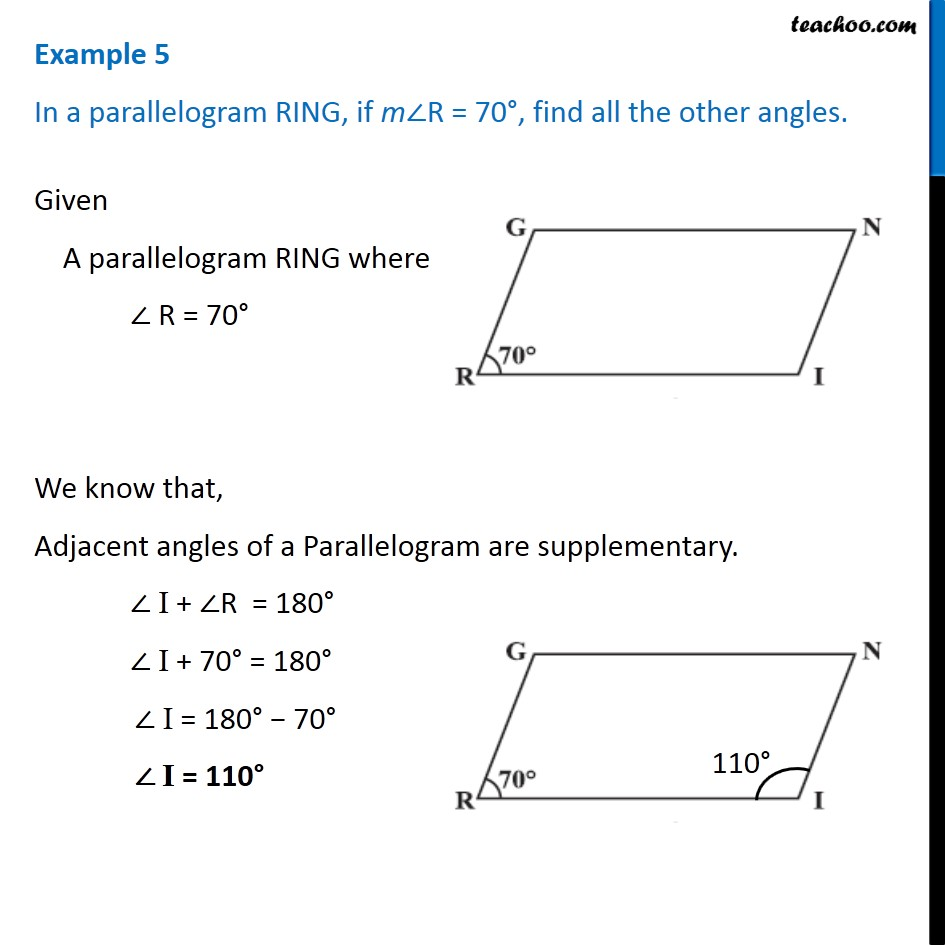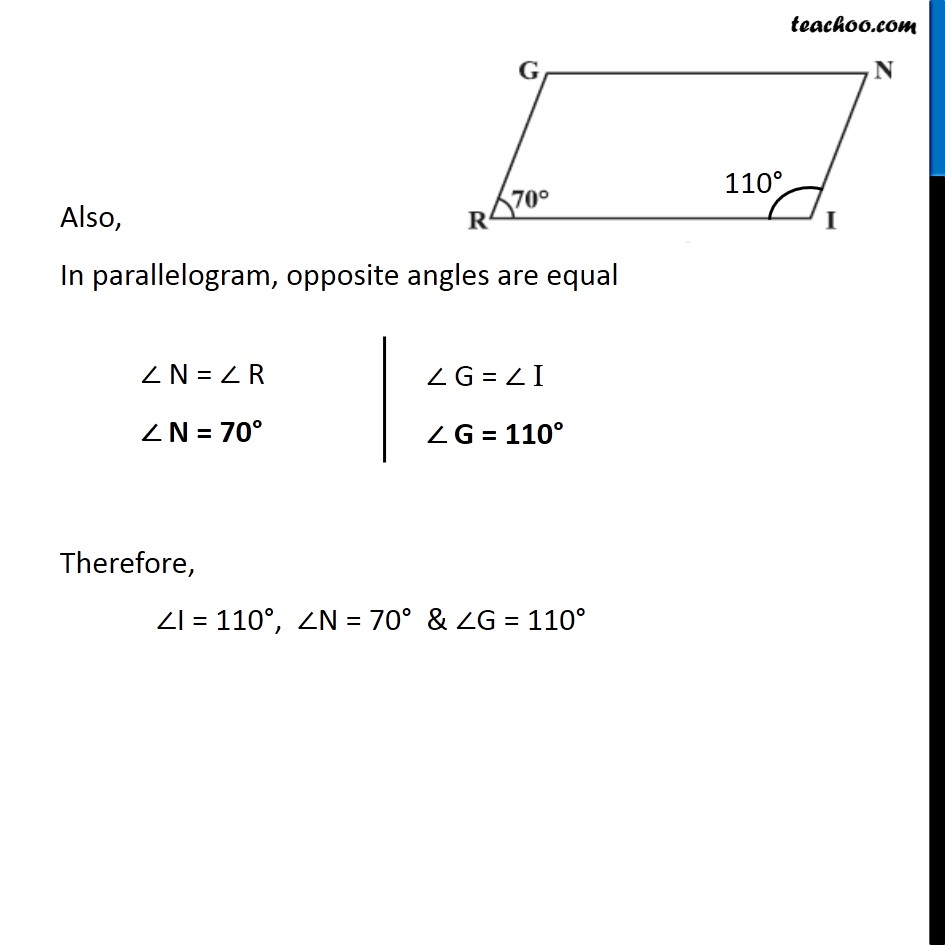Examples

Chapter 3 Class 8 Understanding Quadrilaterals
Serial order wiseLearn in your speed, with individual attention - Teachoo Maths 1-on-1 Class

### Transcript

Example 5 In a parallelogram RING, if m∠R = 70°, find all the other angles. Given A parallelogram RING where ∠ R = 70° We know that, Adjacent angles of a Parallelogram are supplementary. ∠ I + ∠R = 180° ∠ I + 70° = 180° ∠ I = 180° − 70° ∠ I = 110° Also, In parallelogram, opposite angles are equal ∠ N = ∠ R ∠ N = 70° ∠ G = ∠ I ∠ G = 110° Therefore, ∠I = 110°, ∠N = 70° & ∠G = 110°